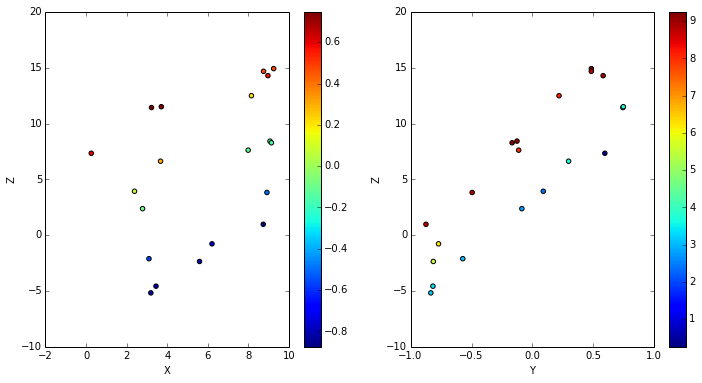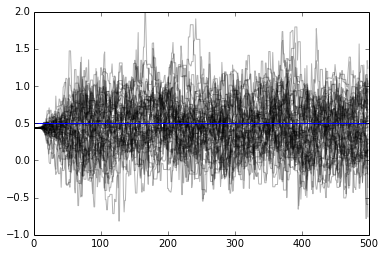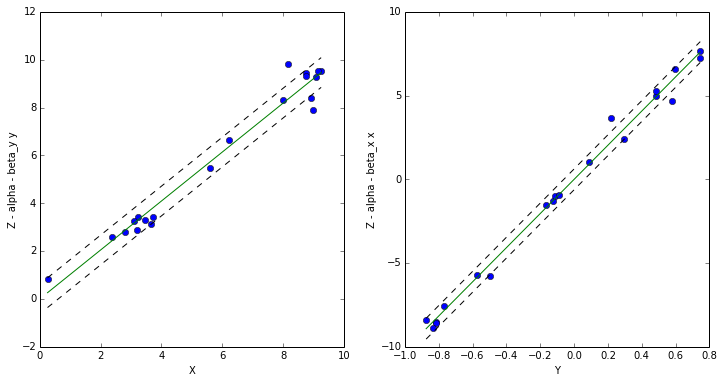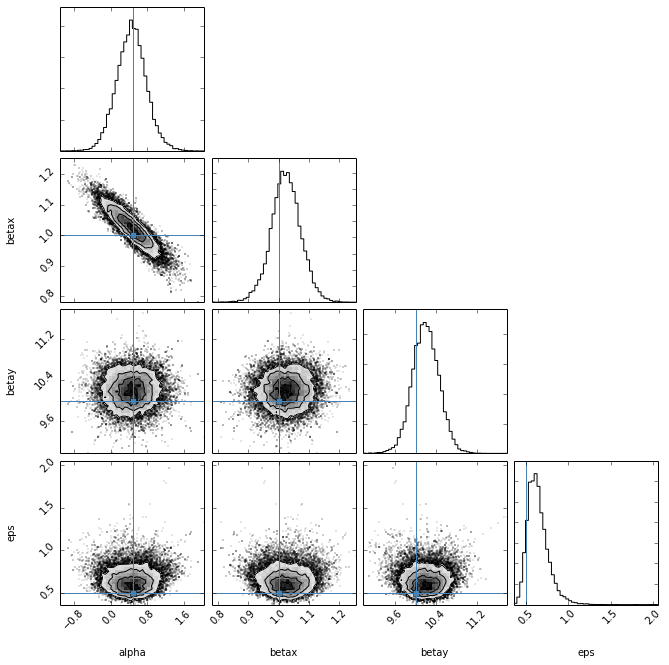Emcee Example¶

emcee is a python module that implements a very cool MCMC sampling algorithm cample an ensemble sampler. In order to more efficiently sample the parameter space, many samplers (called walkers) run in parallel and periodically exchange states. emcee is available from this website:

http://dan.iel.fm/emcee/current/

You can also install via pip or easy_install.

Similar to other examples, we start by making some fake data. In this case, we're going to do have a linear regression model with two predictor variables (x,y) and one outcome variable (z). The relation between them is: $Z_n = \alpha + \beta_x x_n + \beta_y y_n + \epsilon$ where $\epsilon$ is a Gaussian noise term.

In :
from numpy import *
Nobs = 20
x_true = random.uniform(0,10, size=Nobs)
y_true = random.uniform(-1,1, size=Nobs)
alpha_true = 0.5
beta_x_true = 1.0
beta_y_true = 10.0
eps_true = 0.5
z_true = alpha_true + beta_x_true*x_true + beta_y_true*y_true
z_obs = z_true + random.normal(0, eps_true, size=Nobs)

We can plot the data through two diferent slices of the data. We'll color code each point by it's value of the other predictor to see the trend in both cases.
In :
%matplotlib inline
from matplotlib import pyplot as plt
plt.figure(figsize=(12,6))
plt.subplot(1,2,1)
plt.scatter(x_true, z_obs, c=y_true, marker='o')
plt.colorbar()
plt.xlabel('X')
plt.ylabel('Z')
plt.subplot(1,2,2)
plt.scatter(y_true, z_obs, c=x_true, marker='o')
plt.colorbar()
plt.xlabel('Y')
plt.ylabel('Z')

Out:
<matplotlib.text.Text at 0x110ed2590>The big difference between emcee and PyStan and pymc is that the module is all about the sampler and doesn't give you any build-in distributions. You have to write the entire probability function yourself. Following the example on emcee's site, we do this by writing log-prior, log-likelihood, and log-probability functions:

In :
def lnprior(p):
# The parameters are stored as a vector of values, so unpack them
alpha,betax,betay,eps = p
# We're using only uniform priors, and only eps has a lower bound
if eps <= 0:
return -inf
return 0

def lnlike(p, x, y, z):
alpha,betax,betay,eps = p
model = alpha + betax*x + betay*y
# the likelihood is sum of the lot of normal distributions
denom = power(eps,2)
lp = -0.5*sum(power((z - model),2)/denom + log(denom) + log(2*pi))
return lp

def lnprob(p, x, y, z):
lp = lnprior(p)
if not isfinite(lp):
return -inf
return lp + lnlike(p, x, y, z)



At this point, the total probability $p\left(\theta|D\right)$ is given by lnprob. You can imagine that as the model gets more and more complicated and hiarchical, the coding will be much more complicated than with PyStan or pymc. Now, the pymc documentation recommends finding the maximum likelihood using scipy's optimize module:

In :
import scipy.optimize as opt
nll = lambda *args: -lnlike(*args)
result = opt.minimize(nll, [alpha_true, beta_x_true, beta_y_true, eps_true],
args=(x_true, y_true, z_obs))
print result['x']

[  0.4384431    1.02765213  10.207828     0.54077146]



Now that we have the maximum likelihood, we must create a number of walkers to sample our parameters space and instantiate each with a slightly different starting point.

In :
Nwalker,Ndim = 50,4
p0 = [result['x']+1.e-4*random.randn(Ndim) for i in range(Nwalker)]


We feed the lnprob and the initial staring points of the Nwalker walkers to the ensemble sampler and let it run. Multiprocessing is built in, so one can take advantage of multiple CPU's to run the walkers in parallel.

In :
import emcee
sampler = emcee.EnsembleSampler(Nwalker,Ndim,lnprob,
args=(x_true,y_true,z_obs))
pos,prob,state = sampler.run_mcmc(p0, 500)


The sampler will now have a chains attribute which is an array with shape (Nwalker,N,Ndim) where N is the number of interations (500 in our inital run). We can plot these traces out to see what's happening. We'll just look at alpha.

In :
res=plot(sampler.chain[:,:,0].T, '-', color='k', alpha=0.3)
axhline(alpha_true, color='blue')

Out:
<matplotlib.lines.Line2D at 0x1125dfb10>As you can see, the walkers start out all bundled together, but then start to explore the parameter space and finally converge on a fixed dispersion around a value close to the true value (in blue). We can run the sampler for longer. To do this, we reset the sampler (to get rid of the previous chain) and start where the sampler left off (pos).

In :
sampler.reset()
pos,prob,state = sampler.run_mcmc(pos, 1000)


As well as chain, the sampler has a flatchain attribute which simply concatenates all the walkers' chains into one. So the array shape will be (N, Ndim). We compute the medians and plot the results of the model.

In :
m_alpha,m_betax,m_betay,m_eps = median(sampler.flatchain, axis=0)

plt.figure(figsize=(12,6))
plt.subplot(1,2,1)
plt.plot(x_true, z_obs-m_alpha-m_betay*y_true, 'o')
plt.xlabel('X')
plt.ylabel('Z - alpha - beta_y y')
# Now plot the model
xx = array([x_true.min(), x_true.max()])
plt.plot(xx, xx*m_betax)
plt.plot(xx, xx*m_betax + m_eps, '--', color='k')
plt.plot(xx, xx*m_betax - m_eps, '--', color='k')
plt.subplot(1,2,2)
plt.plot(y_true, z_obs-m_alpha-m_betax*x_true, 'o')
plt.xlabel('Y')
plt.ylabel('Z - alpha - beta_x x')
yy = array([y_true.min(), y_true.max()])
plt.plot(yy, yy*m_betay)
plt.plot(yy, yy*m_betay + m_eps, '--', color='k')
plt.plot(yy, yy*m_betay - m_eps, '--', color='k')

Out:
[<matplotlib.lines.Line2D at 0x1158b8ed0>]If you have the triangle_plot plotting package, it's an easy way to look at the covariance of the various parameters. For this to work, we need the Markov chains in a 2D array indexed by [i,p], but that's already how the flatchain array is indexed, so we only have to feed it in.

In :
import triangle
tmp = triangle.corner(sampler.flatchain, labels=['alpha','betax','betay','eps'],
truths=[alpha_true, beta_x_true, beta_y_true, eps_true])